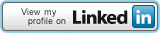## Connect Me## Pages

### WAP to enter a string and print how many letter “P” are in the string. (If input is Pineapple, answer is 3)

DECLARE SUB TOTAL(P\$)
CLS
INPUT "ENTER A STRING"; P\$
CALL TOTAL(P\$)
END

SUB TOTAL(P\$)
P\$=UCASE\$(P\$)
FOR I = 1 TO LEN(P\$)
IF MID\$(P\$,I,1)="P" THEN C=C+1
NEXT I
PRINT "TOTAL NUMBER OF LETTER P IS "; C
END SUB

Same program using more number of lines (line with red color added for more elaboration)

DECLARE SUB TOTAL(P\$)
CLS
INPUT "ENTER A STRING"; P\$
CALL TOTAL(P\$)
END

SUB TOTAL(P\$)
Q\$=UCASE\$(P\$)
L-LEN(Q\$)
FOR I = 1 TO L
R\$=MID\$(Q\$,I,1)
IF R\$="P" THEN C=C+1
NEXT I
PRINT "TOTAL NUMBER OF LETTER P IS "; C
END SUB

Ą